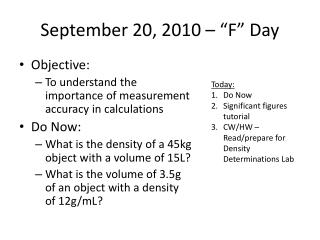DownloadDownload PresentationSeptember 20, 2010 – “F” Day

# September 20, 2010 – “F” Day

Télécharger la présentation## September 20, 2010 – “F” Day

- - - - - - - - - - - - - - - - - - - - - - - - - - - E N D - - - - - - - - - - - - - - - - - - - - - - - - - - -
##### Presentation Transcript

1. September 20, 2010 – “F” Day • Objective: • To understand the importance of measurement accuracy in calculations • Do Now: • What is the density of a 45kg object with a volume of 15L? • What is the volume of 3.5g of an object with a density of 12g/mL? Today: Do Now Significant figures tutorial CW/HW – Read/prepare for Density Determinations Lab

2. September 21, 2010 – “A” Day • Objective: • To understand the importance of measurement accuracy in calculations • Do Now: • What are significant figures? Why are they important? Today: Do Now Go over significant figures notes/problems CW/HW – Read/prepare for Density Determinations Lab

3. Significant Figures Tutorial • Go to http://moourl.com/sigfig • Complete the tutorial and take DETAILED notes • Complete Significant Figures Practice Problems • HW – Read/Prepare for Density Determinations Lab

4. Significant Figures • All measurements are approximations--no measuring device can give perfect measurements without experimental uncertainty • By convention, a mass measured to 13.2 g is said to have an absolute uncertainty of 0.1 g and is said to have been measured to the nearest 0.1 g. • In other words, we are somewhat uncertain about that last digit —it could be a "2"; then again, it could be a "1" or a "3". A mass of 13.20 g indicates an absolute uncertainty of 0.01 g…

5. What is a "significant figure”? • The number of significant figures in a result is simply the number of figures that are known with some degree of reliability. • The number 13.2 is said to have 3 significant figures. • The number 13.20 is said to have 4 significant figures.

6. Rules for deciding the number of significant figures: • (1) All nonzero digits are significant: • 1.234 g has 4 significant figures, • 1.2 g has 2 significant figures. • (2) Zeroes between nonzero digits are significant: • 1002 kg has 4 significant figures, • 3.07 mL has 3 significant figures.

7. Rules Continued • (3) Leading zeros to the left of the first nonzero digits are not significant; such zeroes merely indicate the position of the decimal point: • 0.001 oC has only 1 significant figure, • 0.012 g has 2 significant figures. • (4) Trailing zeroes that are also to the right of a decimal point in a number are significant: • 0.0230 mL has 3 significant figures • 0.20 g has 2 significant figures.

8. Last Rule • (5) When a number ends in zeroes that are not to the right of a decimal point, the zeroes are not significant: • 190 miles has only 2 significant figures, • 50,600 calories has only 3 significant figures. This last rule is a major reason why scientists use scientific notation! • 5.06 × 104 calories (3 significant figures) • 5.060 × 104 calories (4 significant figures), or • 5.0600 × 104 calories (5 significant figures)

9. Multiplying and Dividing • Answers must contain the same number of significant figures as in the number with the least amount of significant figures • Try This: